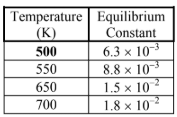# Problem: The table below lists the equilibrium concentrations for H 2(g), I2(g), and HI(g) in the given reaction.H2 (g) + I2 (g) ⇌ 2HI (g)Is the reaction is exothermic or endothermic?

###### FREE Expert Solution

We’re being asked to determine if the reaction is exothermic or endothermic using the data provided

H(g) + I(g) ⇌ 2HI (g)According to Le Chatelier’s Principle, if a system (chemical reaction) is at equilibrium and we disturb it, then the system will readjust to maintain its equilibrium state

94% (123 ratings)###### Problem Details

The table below lists the equilibrium concentrations for H 2(g), I2(g), and HI(g) in the given reaction.

H(g) + I(g) ⇌ 2HI (g)Is the reaction is exothermic or endothermic?